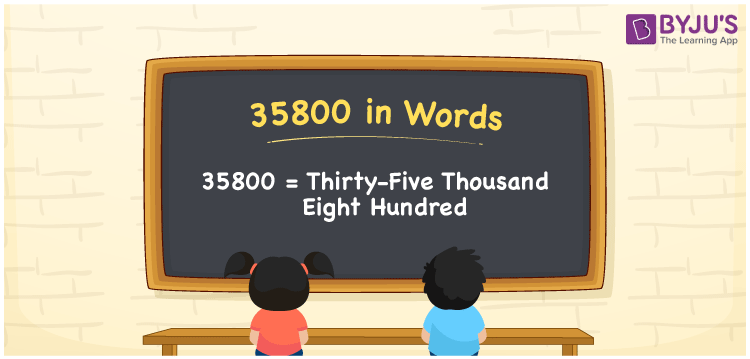# 35800 in Words

The number 35800 in words is represented by Thirty-Five Thousand Eight Hundred. For instance, Siya purchased a golden bracelet worth Rs. 35800, then you can say, “Siya purchased a golden bracelet worth Rupees Thirty-Five Thousand Eight Hundred”. 35800 is a cardinal number as it depicts a certain amount. Let’s learn how to transform the number 35800 into words using a place value method in this article.

 35800 in Words Thirty-Five Thousand Eight Hundred Thirty-Five Thousand Eight Hundred in numerical form 35800

## 35800 in English Words

In Maths, we generally write the numbers in words using the letters of the English alphabet. Therefore, the number 35800 in words is written as Thirty-Five Thousand Eight Hundred.## How to Write 35800 in Words?

We can convert the number 35800 into words by finding the position of each digit using the place value system. The place value chart for the number 35800 is shown below.

 Ten-Thousands Thousands Hundreds Tens Ones 3 5 8 0 0

Hence, we can write the expanded form as:

3 x Ten Thousand + 5 x Thousand + 8 x Hundred + 0 x Ten + 0 x One

= 3 x 10000 + 5 x 1000 + 8 x 100 + 0 x 10 + 0 x 1

= 30000 + 5000 + 800 + 0 + 0

= 30000 + 5000 + 800

= 35800

= Thirty-Five Thousand Eight Hundred

Therefore, 35800 in words is written as Thirty-Five Thousand Eight Hundred

Interesting way of writing 35800 in words

3 = Three

35 = Thirty-Five

358 = Three Hundred and Fifty-Eight

3580 = Three Thousand Five Hundred Eighty

35800 = Thirty-Five Thousand Eight Hundred

Thus, the word form of the number 35800 is Thirty-Five Thousand Eight Hundred

35800 is a natural number that is one less than 35801 and one greater than 35799

• 35800 in words – Thirty-Five Thousand Eight Hundred
• Is 35800 an odd number? – No
• Is 35800 an even number? – Yes
• Is 35800 a perfect square number? – No
• Is 35800 a perfect cube number? – No
• Is 35800 a prime number? – No
• Is 35800 a composite number? – Yes

## Frequently Asked Questions on 35800 in Words

Q1

### What is 35800 in words?

35800 in words is Thirty-Five Thousand Eight Hundred.
Q2

### Simplify 30000 + 5800, and express in words.

Simplifying 30000 + 5800, we get 35800. Therefore, the number 35800 in words is Thirty-Five Thousand Eight Hundred.
Q3

### Write Thirty-Five Thousand Eight Hundred in numbers.

Thirty-Five Thousand Eight Hundred in numbers is 35800.